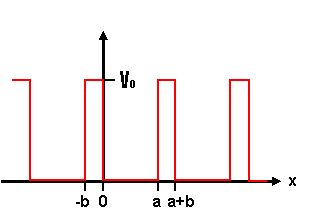# Energy Bands: Kronig-Penney Model

In Kronig-Penney Model we seek solutions for time independent Scrödinger equation inside and outside of the periodic potential.for 0 < x < a;​
V(x) =
V0 for -b < x < 0​

My question is why solution of the KP Scrödinger equation in the region of $$-b < x < 0$$ doesnot have complex exponentials as in the region of $$0 < x < a$$ ?

## Answers and Replies

In this region we have
$$\frac{{d^2 \psi }}{{dx^2 }} = k^2 \psi,$$

where

$$k^2 = \frac{{2m\left( {V_0 - E} \right)}}{{\hbar ^2 }}.$$
The solution to this 2nd order ODE is a linear combination of exponentials (with real arguments). If you think about it physically, the wavefunction will also decay in this area, which can only happen if the argument of the exponential is real.

In this region we have
$$\frac{{d^2 \psi }}{{dx^2 }} = k^2 \psi,$$

where

$$k^2 = \frac{{2m\left( {V_0 - E} \right)}}{{\hbar ^2 }}.$$
The solution to this 2nd order ODE is a linear combination of exponentials.

OK, so what about first region?

$$\frac{{d^2 \psi }}{{dx^2 }} = q^2 \psi,$$

where
$$q^2 = \frac{{2m\left( {E} \right)}}{{\hbar ^2 }}.$$

Isn't it also 2.nd order ODE ?

In the region with V=0 we have
$$\frac{{d^2 \psi }}{{dx^2 }} = -\hat k^2 \psi,$$

where

$$\hat k^2 = \frac{{2m\left( {E} \right)}}{{\hbar ^2 }}.$$

Solutions are travelling waves (going left and right).

Oh i see, I had some conceptual errors on writing the wave eq.
Thanks!
Torehan

I know that this topic doesn't take much attention of most of you as there are more interesting topics about paradoxes of physics but I need a little bit guidance about reproducing the band structure diagrams shown in Kittel , 170p in 8th Edition :)

So we have different wave solution for V0 region and 0 region,

$$\psi_{1}(x)=Ae^{ikx}+Be^{-ikx}\ \ \ \ \ \ \ for \ \ \ \ \ \ \ \ 0<x<a$$

$$\psi_{2}(x)=Ce^{qx}+De^{-qx}\ \ \ \ \ \ \ for \ \ \ \ \ \ \ \ \-b<x<0$$

After we apply the boundary conditions (usual QM boundary condiions in in square well potentials ),

$$\psi_{1}(0) = \psi_{2}(0)$$ and $$\psi_{1}'(0) = \psi_{2}'(0)$$

$$\psi_{1}(a) = \psi_{2}(-b)$$ and $$\psi_{1}'(a) = \psi_{2}'(-b)$$

Then, we have four equations

$$A+B=C+D$$

$$ik(A-B)=q(C-D)$$

$$Ae^{ika}+Be^{-ika}=(Ce^{-qb}+De^{qb})e^{i\alpha(a+b)}$$

$$ik(Ae^{ika}-Be^{-ika})=q(Ce^{-qb}-De^{qb})e^{i\alpha(a+b)}$$

Determinant of the coefficients of this equation system mush vanish to have solutions. Than determinant yields,

$$([q^{2}-k^{2}]/2qk) sinh(qb)sin(ka) + cosh(qb)cosh(ka) = cos (\alpha(a+b))$$

Finally my issues
• How can I define the allowed and forbidden energy values?
• to be continued...

$$e^{i\alpha(a+b)}$$ ---->The term $$\alpha$$ came from Bloch's Theorem and (a+b) came from application of transitional symmetry operation to Bloch form wavefunction!

Last edited: